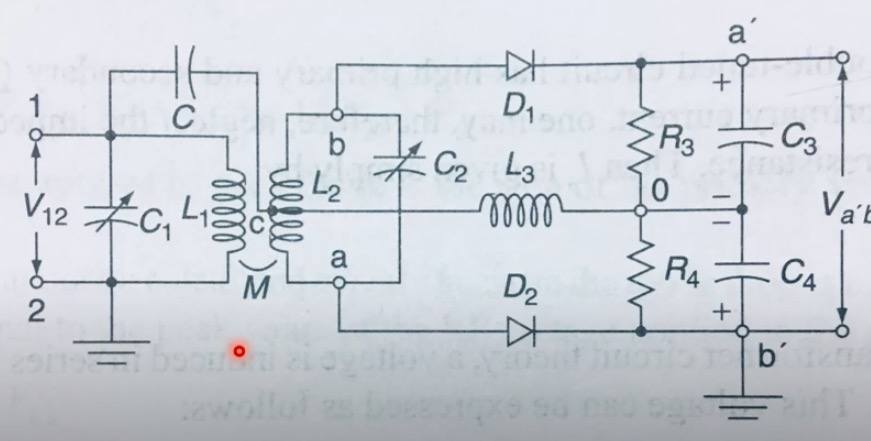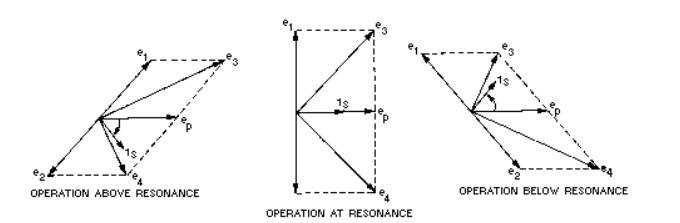# Finding the resonant frequency of this "discriminator" demod circuit.

TL;DR Summary
I'm trying to find the practical resonant frequency and frequency response plots for this circuit.This is the FM demodulation "discriminator" circuit. It is one of the simplest analog demod setups one could think of. An RF (FM) voltage signal is applied at V12 and the voltage at ' a' ' is supposed to contain the AF signal in its amplitude.

The idea behind the circuit is that it resonates at the RF carrier frequency (high), and as the frequency dips above and below the carrier frequency, the frequency response of the circuit dips above and below in voltage amplitude. The resonating L1 coil passes its voltage to the tapped L2. The voltage at ' a' ' and ' b' ' are the vector sum of the voltages across L3 and the halves of L2. But taking loops from L3 shows that they are the sum and difference between L3 and half of the voltage across L2 respectively. When V12 is above or below the carrier frequency, and therefore the circuit is below resonance, the current and voltage through L1 are no longer in phase since the impedance is not purely resistive. L2 voltage is at a 90 deg phase shift from the current (emf = L di/dt), and the voltage across L3 is in phase with the voltage across L1 since they are effectively in parallel (C and C4 are have low reactance). So there is a phase difference between the L2 and L3 voltages that is not 90 degrees. Drawing a phasor diagram helps:Where e1 and e2 are opposite polarity voltage drops through L2 from the centre tap; ep is the voltage across L3 and L1 (same, same); e3 and e4 are vector sums of e1 with e3 and e2 with e3, and 1_s or "i_s" is the current through L1.

The voltage V_a'b' is either the difference or the sum of e3 and e4, I'm not sure. I think the circuit would work if it was the sum - which is twice e3, and varies in amplitude with the frequency response of the circuit.

The RC filter has a time constant much higher than the carrier frequency, but low enough to pass AF (20 -> 20000 Hz). Then as the frequency varies at AF time scales, the voltage amplitude varies at AF time scales due to frequency response and the AF voltage oscillations are passed through to V_ab.

I kind of understand the circuit conceptually, although some beliefs might be dodgy like whether the AF output is the sum or difference of e3 and e4.

I want to write an equation for the frequency response of the elements in the circuit. Is this feasible?

I tried to divide the circuit into a CLC (with a resistor) series filter and a band stop filter at the primary of the transformer. I assume R3 and R4 to be asymptotically high. I presume we need to imagine there are resistances in some places - but where? and in parallel or series and how much? And does the secondary impedance need to be reflected? Would this affect the resonant frequency?

Does anyone have any ideas how to find the frequency at which the LC tank with some assumed resistance resonates, as well as the CLC filter of C, L3 and C4 with some assumed resistance? Or if those questions are the wrong questions, does anyone know how this circuit resonates? And in particular, how to plot the frequency response.

Thanks.

The frequency response is of the type shown on page 12 of the following link.

It consists of an almost linear slope across the signal passband then falling away rapidly outside this.
The two windings are loosely coupled in order to obtain 90 deg phase shift between them. In other words, the coefficient of coupling (k) is less than 1. This can be obtained by making the mutual inductance less than (L2 k)/Q. The Q of each winding is decided by the losses and the loads across them. For the primary it is the output impedance of the preceding amplifier and for the seconday it is the diode load resistance. As each diode conducts for half the time, each resistor carries current for half the time, but overall the tuned circuit sees one resistor all the time. The Q of the circuit is therefore R3/XC2 or R3/XL2.

The Q of the circuits are chosen so that the peaks are far enough apart to encompass the entire received signal. This bandwidth is greater than the deviation. Approximately speaking I believe this means a Q about equal to the percentage bandwidth. If Q is too low we have poor sensitivity and if Q is too high we have distortion.

L3 is a choke, whose function is to allow the DC diode load current to pass but not radio frequencies, so its reactance should be higher than the load resistors at the carrier frequency. C3 and C4 are to smooth the output, so have low impedance at carrier frequency but high impedance at audio frequency, compared to R3 and R4. The coupling capacitor C seems to have no function other than to block DC.

Last edited by a moderator:
•Klystron and berkeman
The frequency response is of the type shown on page 12 of the following link.

It consists of an almost linear slope across the signal passband then falling away rapidly outside this.
The two windings are loosely coupled in order to obtain 90 deg phase shift between them. In other words, the coefficient of coupling (k) is less than 1. This can be obtained by making the mutual inductance less than (L2 k)/Q. The Q of each winding is decided by the losses and the loads across them. For the primary it is the output impedance of the preceding amplifier and for the seconday it is the diode load resistance. As each diode conducts for half the time, each resistor carries current for half the time, but overall the tuned circuit sees one resistor all the time. The Q of the circuit is therefore R3/XC2 or R3/XL2.

The Q of the circuits are chosen so that the peaks are far enough apart to encompass the entire received signal. This bandwidth is greater than the deviation. Approximately speaking I believe this means a Q about equal to the percentage bandwidth. If Q is too low we have poor sensitivity and if Q is too high we have distortion.

L3 is a choke, whose function is to allow the DC diode load current to pass but not radio frequencies, so its reactance should be higher than the load resistors at the carrier frequency. C3 and C4 are to smooth the output, so have low impedance at carrier frequency but high impedance at audio frequency, compared to R3 and R4. The coupling capacitor C seems to have no function other than to block DC.

This great overview has given me a better insight into the exact intended functions, such as that of the L3 choke, of the circuit elements, as well as into some important considerations in choosing component impedances. Thank you very much.

•berkeman